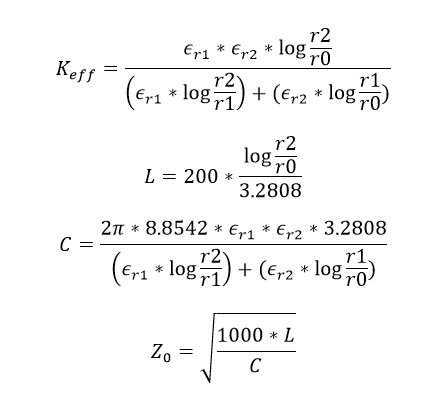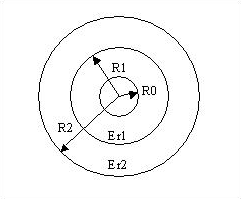# Dual Dielectric Coax Calculator

This CalcTown calculator calculates the capacitance, inductance, impedance and various other parameters related to a dual dielectric coaxial cable.

When a coaxial cable is constructed using two concentric dielectrics as shown above, the wave propagation is no longer purely transverse-electromagnetic (TEM), as in the single-dielectric coax, but instead is quasi-TEM, like microstrip or CPW and can suffer from dispersion. But the "non-TEM-ness" is very slight indeed. In the most general terms the characteristic impedance and effective dielectric constant are very slight functions of frequency.

#### Result

pF/ft
nH/ft
ΩWhere,

r0 = radius of the inner layer

r1 = radius of the outer layer

r2 = external radius of the cable

εr1 = relative permittivity of inner layer

εr2 = relative permittivity of outer layer

Z0 = impedance per unit length of the cable

C = capacitance per unit length of the cable

L = inductance per unit length of the cable

To calculate the static capacitance between the inner and outer conductors, we imagine a zero-thickness conducting cylinder placed inside the cable, at the interface between the two dielectrics. Since all of the electric field components are already perpendicular to this interface, this does not violate the boundary conditions of the initial problem. With this conducting cylinder in place, two capacitance values can now be calculated:

1) the capacitance (per meter length of cable) between the inner conductor and the cylinder; and

2) the capacitance between the cylinder and the outer conductor.

The capacitance per meter of cable between the inner and outer conductors is then the series combination of the above two capacitance values.